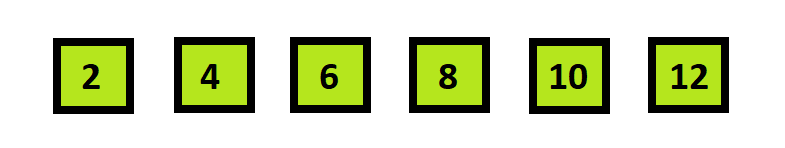×

Search anything:

# Interpolation Search [EXPLAINED]

#### Algorithms Search AlgorithmsIn this article, we have explained the concept of Interpolation Search and analyzed the performance of interpolation search in terms of time and space complexity.

# What is Interpolation Search?

Interpolation search is basically an improvement over binary search. In interpolation search we check at different postitions based on the value of element being searched using a formula , that is :

``````pos= start + (((double)  (end-start) / (a[end]-a[start])) * (key-a[start]))
``````

where,

• pos = position of element
• a = sorted array of elements
• start = starting index of array
• end = last index of array

Note : We have used (double) in the equation because for the division part we need the decimal part to multiply it with (key- a[start]) and get the pos value in (int) type, otherwise the answer may turnout to be 0 and we will get wrong answer.

• For interpolation search to work efficiently the array must be sorted and uniformly distributed otherwise the time complexity may go upto O(n) which is worse than binary search.
• Interpolation search works best for large sorted arrays with uniformly distributed values with average time complexity of O(log(logn)).

# Code :

``````#include<bits/stdc++.h>
using namespace std;

int interpolation_search(int n,int a[],int key)
{
int start=0,end=n-1,pos;
while(start<=end && key<=a[end] && key>=a[start])
{
pos=start+(((double)(end-start)/(a[end]-a[start]))*(key-a[start]));

if(key==a[pos])
return pos;

if(key>a[pos])
start=pos+1;

if(key<a[pos])
end=pos-1;
}
return -1;
}
``````

# Analysis :

## Space Complexity :

Space complexity of Interpolation search is always O(1), as we are not using any extra significant memory which is dependent on input.

## Best case Time complexity : O(1)

Time complexity of this search is highly dependent on uniformity of elements in the array.
We get O(1) time complexity when the key value we need to search for is in the middle of the array, then we get the best time complexity.

We have this uniformly distributed sorted array :In this if the key element is middle element or close to middle element we will get the output in constant time, but this is a very small array so we will get constant time answer for all the elements if we give as key.

## Average case Time Complexity : O(log(logn))

Interpolation search when applied on sorted and uniformly distributed array gives Average Time Complexity of O(log(logn)) and thus is a better searching algorithm when a uniformly distributed array is given.

## Worst case Time Complexity : O(n)

Worst case occurs when the elements in the array are exponentially distributed, example : arr = { 1, 2, 3, 4, 5, 6, 7, 8, 9, 1000000}
In this array if we want to search for element = 9,

We will get first pos value as 0, beacuse of the divison in formula ( end-start / arr[end]-arr[start ) = (10-0)/(1000000-9) = 0.000100009

And anything multiplied with this number would be 0 so, the loop will run close to n times and hence contributing towards the worst case time complexity of O(n).#### Ue Kiao, PhD

Ue Kiao is a Technical Author and Software Developer with B. Sc in Computer Science at National Taiwan University and PhD in Algorithms at Tokyo Institute of Technology | Researcher at TaoBao

Interpolation Search [EXPLAINED]